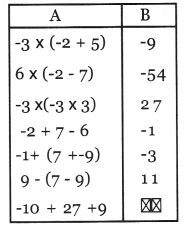# Kerala Syllabus 8th Standard Maths Solutions Chapter 9 Negative Numbers

## Kerala State Syllabus 8th Standard Maths Solutions Chapter 9 Negative Numbers

### Negative Numbers Text Book Questions and Answers

Textbook Page No 165

Negative Numbers Class 8 Kerala Syllabus Question 1.
Using the principles above, compute the following:
i. 5 – 10
ii. -10 + 5
iii. -5 – 10
iv. -5 – 5
vi. $$-\frac{1}{2}+1 \frac{1}{2}$$
vii. $$-\frac{1}{2}-1 \frac{1}{2}$$
viii. $$-\frac{1}{2}+\frac{1}{2}$$
Solution:
i. 5 – 10 = – (10 – 5) = -5
(since for x – y = -(y – x ))

ii. – 10 + 5 = 5 – 10 = – (10 – 5) = -5
(since for -x + y = y – x and x – y = – (y – x))

iii. -5 – 10 = – (5 + 10 ) = -15
(since for – x – y = -(x + y))

iv. -5 – 5 = – (5 + 5) = -10
(since for -x – y = -(x + y))

v. -5 + 5 = 5 – 5 = 0
(since for -x + y = y – x)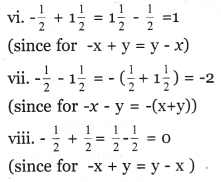Kerala Syllabus 8th Standard Maths Notes Pdf Question 2.
Take as x different positive numbers, negative numbers and zero, and compute x + 1, x – 1, 1 – x. Check whether the equations below hold for all numbers.
i. (1 + x) + (1 – x) = 2
ii. x – (x – 1) = 1
iii. 1 – x = -(x – 1)
Solution:
If x = 1
x + 1 = 1 + 1 = 2
x – 1 = 1 – 1 = 0
1 – x = 1 – 1 = 0
If x = 2
x + 1 = 2 + 1 = 3
x – 1 = 2 – 1 = 1
1 – x = 1 – 2 – 1
If x = o
x + 1 = 0 + 1
x – 1 = 0 – 1 = -1
1 – x = 1 – 0 = 1
If x =-1
x + 1 = -1 + 1 = 1 – 1 = 0
x – 1 = -1 – 1 = -2
1 – x = 1 – (-1) = 1 + 1 = 2
If x = -2
x + 1 = -2 + 1 = -1
x – 1 = -2 – 1 = -3
1 – x = 1 – (-2) = 1 + 2 = 3

i. (1 + x) + (1 – x)
In x = 1, (1 + x) + (1 – x) = 2 + 0 = 2
In x = 2, (1 + x) + (1 – x) = 3 + (-1) = 3 – 1 = 2
In x = o, (1 + x) + (1 – x) = 1 + 1 = 2
In x = -1, (1 + x) + (1 – x) = 0 + 2 = 2
In x – 2, (1 + x) + (1 – x) – 1 + 3 = 3 – 1 = 2
(1 + x) + (1 – x) = 2 , for all values of x

ii. x – (x – 1)
In x = 1, x – (x – 1) = 1 – 0 = 1
In x = 2, x – (x – 1) = 2 – 1 = 1
In x = o, x – (x – 1) = 0 – (-1) = 1
In x = -1, x – (x – 1) = -1 – (-2) = -1 + 2 = 1
In x =-2, x – (x – 1) = -2 – (-3) = -2 + 3 = 1
x – (x – 1) = 1, for all values of x

iii. 1 – x
In x = 1, 1 – x = o = -(x – 1)
In x = 2, 1 – x = -1 = -(1) = -(x – 1)
In x = o, 1 – x = 1 = -(-1) = -(x – 1)
In x = -1, 1 – x = 2 = -(-2) = -(x – 1)
In x = -2, 1 – x = 3 = -(-3) = -(x – 1)
1 – x = -(x – 1), for all values of x

Hsslive Guru Maths 8th Standard Kerala Syllabus Question 3.
Taking different numbers as x, y and compute x + y, x – y. Check whether the following hold for all kinds of numbers.
i. (x + y) – x = y
ii. (x + y) – y = x
iii. (x – y) + y = x
Solution:
If we take x = 6 and y = 2, x + y = 6 + 2 = 8 and x – y = 6 – 2 = 4
If x = -6 and y = 2, x + y = -6 + 2 = -4 and x – y = -6 – 2 = -8
If x = 6 and y = -2, x + y = 6 + (-2) = 4
and x – y = 6 – (-2) = 8
If x = -6 and y = -2, x + y = (-6) + (-2)
= -8 and x – y = (-6) – (-2 ) = -4

i. (x + y) – x
If x = 6 and y = -2, (x + y) – x
= 8 – 6 = 2 = y
If x = -6 and y = 2, (x + y) – x
= -4 – (-6) = -4 + 6 = 2 = y
If x = 6 and y = -2, (x + y) – x
= 4 – 6 = – 2 = y
If x = -6 and y = -2, (x + y) – x
= -8 – (-6) = -8 + 6 = -2 = y
(x + y) – x = y, for all values of x and y

ii. (x + y) – y
If x = 6 and y = 2, (x + y) – y
= 8 – 2 = 6 = x
If x = -6 and y = 2, (x + y) – y
= -4 – 2 = -6 = x
If x = 6 and y = -2, (x + y) – y
= 4 – (-2) = 4 + 2 = 6 = x
If x = -6 and y = -2, (x + y) – y
= -8 – (-2) = -8 + 2 = -6 = x
(x + y) – y = x, for all values of x and y

iii. (x – y) + y
If x = 6 and y = 2, (x – y) + y
= 4 + 2 = 6 = x
If x = -6 and y = 2, (x – y) + y
= -8 + 2 = -6 = x
If x = 6 and y = -2, (x – y) + y
= 8 + (-2) = 8 – 2 = 6 = x
If x = -6 and y =- 2, (x – y) + y
= -4 + (-2) = -6 = x
(x – y) + y = x, for all values of x and y

8th Standard Maths Notes Kerala Syllabus Question 4.
Check whether the equation are identities. Write the patterns got from each, on taking x = 1, 2, 3, 4, 5 and x = -1, -2, -3, -4, -5.
i. -x + (x + 1) = 1
ii. -x + (x + 1) + (x + 2) – (x + 3) = o
iii. -x – (x + 1) + (x + 2) + (x + 3) = 4
Solution:
i) -x + (x + 1) = 1
If x = 1, -x + (x + 1)
= -1 + (1 + 1) = -1 + 2 = 1
If x = 2, -x + (x + 1)
= -2 + (2 + 1) = -2 + 3 = 1
If x = 3, -x + (x + 1)
= -3 + (3 + 1) = -3 + 4 = 1
If x = 4 -x + (x + 1)
= 4 + (4 + 1) = -4 + 5 = 1
If x = 5, -x + (x + 1)
= -5 + (5 + 1) = -5 + 6 = 1
If x = -1, -x + (x + 1)
= -(-1) + (-1 + 1) = 1 +0 = 1
If x = -2, -x + (x + 1)
= -(-2) + (-2 + 1) = 2 + (-1) = 1
If x = -3, -x + (x + 1)
= -(-3) + (-3 + 1) = 3 + (-2) = 1
If x = -4, -x + (x + 1)
= -(-4) + (-4 + 1) = 4 + (-3) = 1
If x = -5, -x + (x + 1)
= -(-5) + (-5 + 1) = 5 + (-4) = 1
It is an identity.

ii. -x + (x + 1) + (x + 2) – (x + 3) = 0
If x = 1, -x + (x + 1) + (x + 2) – (x + 3)
= -1 + (1 + 1) + (1 + 2) – (1 + 3)
= -1 + 2 + 3 – 4 = 0
If x = 2, -x + (x + 1) + (x + 2) – (x + 3)
= -2 + (2 + 1) + (2 + 2) – (2 + 3)
= -2 + 3 + 4 – 5 = 0
If x = 3, -x + (x + 1) + (x + 2) – (x + 3)
= -3 + (3 + 1) + (3 + 2) – (3 + 3)
= -3 + 4 + 5 – 6 = o
If x = 4 -x + (x + 1) + (x + 2) – (x + 3)
= -4 + (4 + 1) + (4 + 2) – (4 + 3)
= -4 + 5 + 6 – 7 = 0
If x = 5, -x + (x + 1) + (x + 2) – (x + 3)
= -5 + (5 + 1) + (5 + 2) – (5 + 3)
= -5 +6 +7 -8= 0
If x = -1, -x + (x + 1) + (x + 2) – (x + 3)
= -(-1) + (-1 + 1) + (-1 + 2) – (-1 + 3)
= 1 + 0 + 1 – 2 = 0
If x = -2, -x + (x + 1) + (x + 2) – (x + 3)
= 2 + (-2 + 1) + (-2 + 2) – (-2 + 3)
= 2 + -1 + 0 – 1 = 0
If x = -3, -x + (x + 1) + (x + 2) – (x + 3)
= 3 + (-3 + 1) + (-3 + 2) – (-3 + 3)
= 3 + -2 + -1 – 0 = 0
If x = -4, -x + (x + 1) + (x + 2) – (x + 3)
= 4 + (-4 + 1) + (-4 + 2) – (-4 + 3)
= 4 + -3 + -2 – (-1) = 0
If x = -5, -x + (x + 1) + (x + 2) – (x + 3)
= 5 + (-5 + 1) +(-5 + 2) – (-5 + 3)
= 5 + -4 + -3 – (-2) = o
It is an identity.

iii. -x – (x + 1) + (x + 2) + (x + 3) = 4
If x = 1, -x – (x +1) + (x + 2) + (x + 3)
= -1 – (1 + 1) + (1 + 2) + (1 + 3)
= -1 – 2 + 3 + 4 = 4
If x = 2, -x – (x + 1) + (x + 2) + (x + 3)
= -2 – (2 + 1) +(2 + 2) + (2 + 3)
= -2 – 3 + 4 + 5 = 4
If x = 3, -x – (x + 1) + (x + 2) + (x + 3)
= -3 – (3 + 1) + (3 + 2) + (2 + 3)
= -3 – 4 + 5 + 6 = 4
If x = 4 -x – (x + 1) + (x + 2) + (x + 3)
= -4 – (4 + 1) + (4 + 2) + (4 + 3)
= -4 – 5 + 6 + 7 = 4
If x = 5, -x – (x + 1) + (x + 2) + (x + 3)
= -5 – (5 + 1) + (5 + 2) +(5 + 3)
= -5 – 6 + 7 + 8 = 4
If x = -1, -x – (x + 1) + (x + 2) + (x + 3)
= -(-1) – (-1 + 1) + (-1 + 2) + (-1 + 3)
= 1 – 0 + 1 + 2 = 4
If x = -2, -x – (x + 1) + (x + 2) + (x + 3)
= 2 – (-2 + 1) + (-2 + 2) + (-2 + 3)
= 2 – (-1) + 0 + 1 = 4
If x = -3, -x – (x + 1) + (x + 2) + (x + 3)
= 3 – (-3 + 1) + (-3 + 2) + (-3 + 3)
= 3 – (-2) + – 1 + 0 = 4
If x = -4, -x – (x + 1) + (x + 2) + (x + 3)
= 4 – (-4 + 1) + (-4 + 2) + (-4 + 3)
= 4 – (-3) + – 2 + (-1) = 4
If x = -5, -x – (x + 1) + (x + 2) + (x + 3)
= 5 – (-5 + 1) + (-5 + 2) + (-5 + 3)
= 5 – (-4) + -3 + (-2) = 4
It is an identity.

Kerala Syllabus 8th Standard Maths Notes Question 5.
Taking different numbers, positive, negative and zero, as x, y, z and compute x + (y + z) and (x + y) + z. Check whether the equation, x + (y + z) = (x + y) + z holds for all these numbers.
Solution: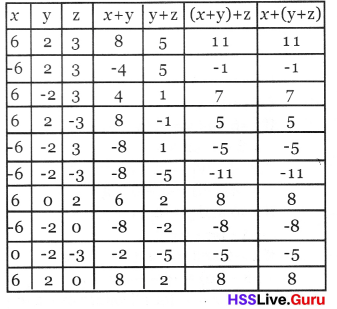Textbook Page No 178

Class 8 Maths Kerala Syllabus Question 6.
Take various positive and negative numbers as x, y, z and compute (x + y) z and xz + yz. Check whether the equation (x + y) z = xz + yz holds for all these.
Solution: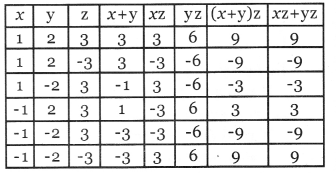Kerala Syllabus 8th Standard Maths Guide Question 7.
In each of the following equations, take x as the given numbers and compute the numbers y.
i. y = x2, x = -5, x = 5
ii. y = x2 + 3x + 2, x = -2
iii. y =x2 + 5x + 4, x = -2, x = -3
iv. y = x3 + 1, x = -1
v. y = x3 + x2 + x + 1
Solution:
i. y = x2 , x = -5 , x = 5
If x =-5, y = x2 = (-5 )2 = -5 × -5 = 25
If x = 5, y = x2 = (5)2 = 5 × 5 = 25

ii. y = x2 + 3x + 2, x = -2
If x = -2, y = x2 + 3x + 2 = (-2)2 + 3x(-2) + 2
= 4 – 6 + 2 = -2 + 2 = 0

iii. y = x2 + 5x + 4, x = -2 , x = -3
If x = -2, y = x2 + 5x + 4 = (-2 )2 + 5x(-2) + 4
= 4 – 10 + 4 = -6 + 4 = -2
If x = -3, y = x2 + 5x + 4 = (-3)2 + 5x(-3) + 4
= 9 – 15 + 4 = -6 + 4 = -2

iv. y = x3 + 1, x = -1
If x = -1, y = x3 + 1 = (-1 )3 + 1
= (-1)(-1)(-1) + 1 = 1 × (-1) + 1 = 0

v. y = x3 + x2 + x + 1, x = -1
If x = -1, y = x3 + x2 + x + 1 = (-1)3 +(-1)2 + (-1) + 1 = -1 + 1 – 1 + 1 = o

Kerala Syllabus 8th Standard Maths Question 8.
For a point starting at a point P and travelling along a straight line, time of travel is taken as t and the distance from P as s. The relation between s and t is found to be s = 12t – 2t2, where distances to the right are taken as positive numbers and to the left as negative numbers.
i. Is the position of the point to the right or left of P, till 6 seconds?
ii. Where is the position at 6 seconds?
iii. After 6 seconds?
(Here it is convenient to write 12t – 2t2 = 2t(6 – t).
Solution:
i. S = 12t – 2t2
Distance to the point when time is 1 second = 12t – 2t2 = 12 × 1 – 2 × 12 = 12 – 2 = 10 m
Distance to the point when time is 5 second = 12t – 2t2 = 12 × 5 – 2 × 52 = 60 – 50 = 10 m
Since the distance to the point till 6 seconds is positive. So the position of the point is on the right of P.
ii. Distance to the point when time is 6 second = 12t – 2t2 = 12 × 6 – 2 × 62 = 72 – 72 = o
At the 6th second, the point is at P.
iii. Distance to the point when time is 7 second (after 6 sec)= 12t – 2t2 = 12 × 7 – 2 × 72
= 84 – 2 × 49 = 84 – 98 = -14 metres
This is a negative number, So the position of the point is on the left of P.

Textbook Page No 179

8th Std Maths Guide Kerala Syllabus Question 9.
Natural numbers, their negatives and zero are together called integers. How many pair of integers are there, satisfying the equation. x2 + y2 = 25?
Solution:
It is convenient to write it as a table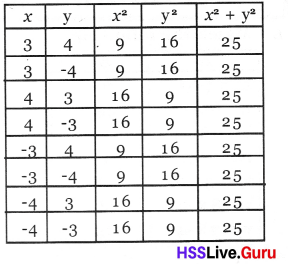Textbook Page No 180

Kerala Syllabus 8th Standard Maths Notes Malayalam Medium Question 10.Solution: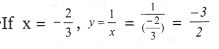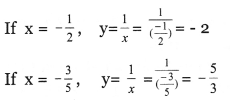Class 8 Kerala Syllabus Maths Question 11.Solution:Class 8 Maths Scert Solutions Kerala Syllabus Question 12.
In the equation $$z=\frac{x}{y}-\frac{y}{x}$$, take x as the numbers given below and calculate the number z.
i. x = 10, y = -5
ii. x = -10, y = 5
iii. x = -10, y = -5
iv. x = 5, y = -10
v. x = -5, y = 10
Solution: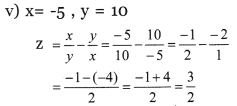Maths Class 8 Kerala Syllabus  Question 1.
Match the following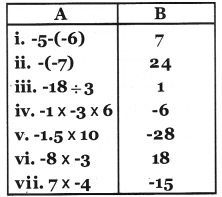Solution: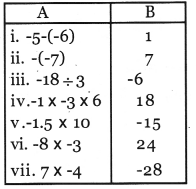8th Class Maths Notes Kerala Syllabus Question 2.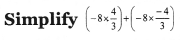Solution: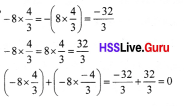Question 3.
Which of the following number is the largest (-1)6, (-1)10, (-1)2, (-1)50
Solution:
If the power of (-1) is even then answer will be 1
If the power of (-1) is odd then answer will be -1
(-1)6 = 1
(-1)10 = 1
(1)2 = 1
(-1)50 = 1
All the given numbers are equal.

Question 4.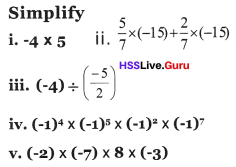Solution: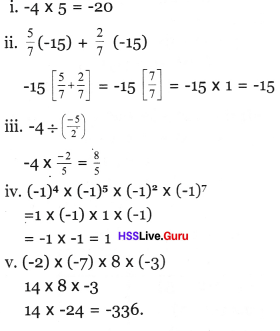Question 5.
Complete the following table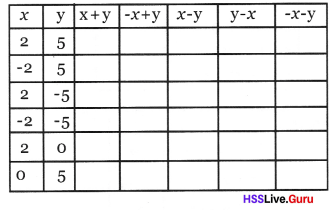Solution:Question 6.
Write whether the answer got on doing the following operations are positive number or negative number.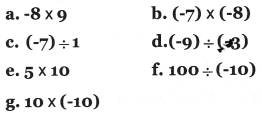Solution: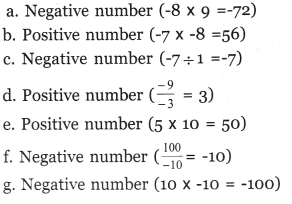Question 7.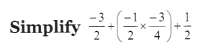Solution: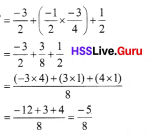Question 8.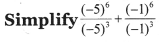Solution:Question 9.
Calculate (-1)10 + (-1)17 + (-1)21 + (-1)26 + (-1)77
Solution:
(-1)10 + (-1)17 + (-1)21 + (-1)26 + (-1)77
= 1 + (-1) + (-1) + 1 + (-1)
= 1 – 1 – 1 + 1 – 1 = 2 – 3 = -1

Question 10.
Simplify [(-4) × (-5)] + [-16 × $$\frac{-1}{2}$$ ]
Solution:
-4 × -5 = 20Question 11.
If x = 8 and y = -3; find the values of x + y, y + x, x – y, y – x, -x – y and – y – x
Solution:
x + y = 8 + -3 = 5
y + x = -3 + 8 = 5
x – y = 8 – (-3) = 8 + 3 = 11
y – x = -3 – 8 = -11
– x – y = -8 – (-3) = -8 + 3 = -5
-y – x = -(-3) – 8 = 3 – 8 = -5

Question 12.
If x = 7, y = -6 and z = -2, find the value of
i. (x + y) + z
ii. x + (y + z)
iii. xyz
iv. (x + y)z
v. xy + xz
Solution:
i. (x + y) + z = (7 + -6) + -2 = 1 – 2 = -1
ii. x + (y + z) = 7 + (-6 + -2)
= 7 – 8 = -1
iii. xyz = 7 × -6 × -2 = 84
iv. (x + y)z = (7 + -6) × -2
= 1 × -2 = -2
v. xy + xz = (7 × -6) + (7 × -2)
= -42 – 14
= -56

Question 13.
Compute y = x2 + 9x – 5, for take x as the given number,
i. x = 5
ii. x = -2
iii. x = o
iv. x = -3
Solution:
i. x = 5
y = x2 + 9x – 5
= 52 + 9 × 5 – 5 = 25 + 45 – 5
= 20 + 45 = 65

ii. x = -2
y = x2 + 9x – 5
=(2)2 + 9 × (-2) -5
= 4 – 18 – 5 = 4 – 23 = – 19

iii. x = 0
y = x2 + 9x – 5
= 0 + 9 × 0 – 5 = -5

iv. x = -3
y = x2 + 9x – 5
= (-3)2 + 9 × (-3) – 5
= 9 – 27 – 5 = 9 – 32 = -23

Question 14.
Find y = x4 + x3 + x2 + x + 1, If x = -1
Solution:
y = x4 + x3 + x2 + x + 1
= (-1)4 + (-1)3 + (-1)2 + (-1) + 1
= 1 + (-1) + 1 + (-1) + 1
= 1 – 1 + 1 – 1 + 1
= 0 + 0 + 1 = 1

Question 15.
Complete the table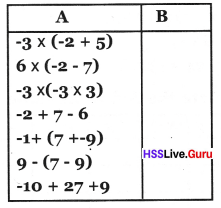Solution: